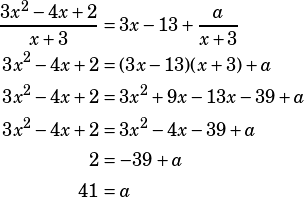Hi Mike,

Can you give me some help with question 10 in the Polynomials section of PWN (pg 149)? I plug in x=-3, per your suggestion, and I get 41 as the answer on the left-hand side of the equation and 3(-3) – 13 + a on the right. That means 41 = -22 + a, or a = 63. What am I missing? Thanks!

Sure! Here’s the question: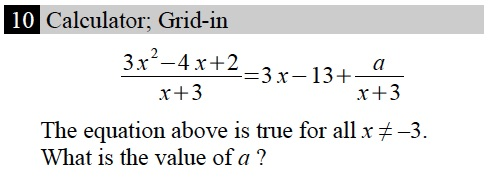The key to this is recognizing that the original equation you’re getting is in quotient-remainder form:is the quotient with a remainder of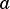. Once you recognize that, you have a few options. You can actually do the division to find the remainder, you can multiply the whole equation by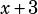and solve for, or you can use the remainder shortcut (since you’re only asked for the remainder in this question) by plugginginto only the original polynomial.

The shortcut: When a polynomialis divided by, where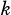is a constant, you can find the remainder simply by finding. In this case, that means we can find the remainder when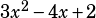is divided bysimply by simplifying, which as you note comes out to 41.

Note that we actually can’t plug inin the original equation: we’d get division by zero on both sides.

I’m not going to do the whole long division here, but because I know that shortcut is confusing, I want to stress that just solving the equation foris also a decent way to go. To begin, multiply everything by.